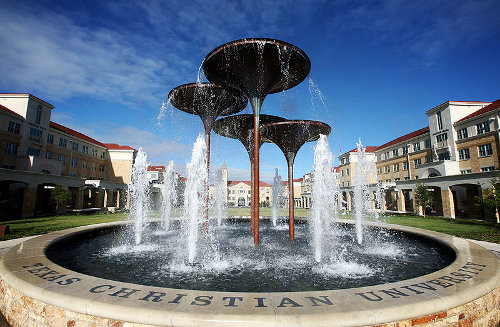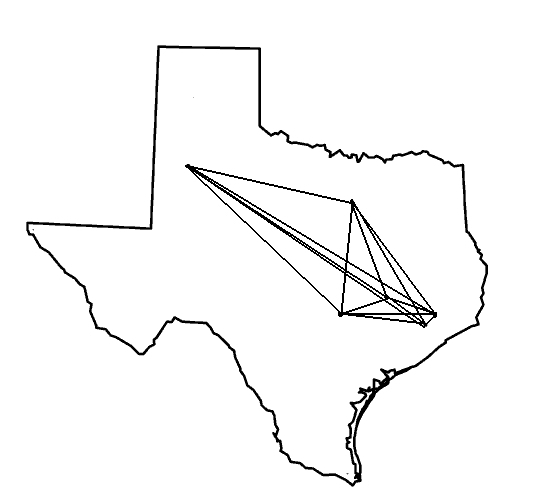# Texas Geometry and Topology Conference

### Texas Christian University Fort Worth, Texas February 19-21, 2016## Schedule and Abstracts

All talks will take place in Tucker Technology Center Room 138. See the campus map for location of Tucker Technology Center.

The full conference schedule can be found here.

## Abstracts of talks:

Christian Bär, University of Potsdam
An Index Theorem for Compact Lorentzian Manifolds with Boundary
Abstract: We show that the Dirac operator on a compact globally hyperbolic Lorentzian spacetime with spacelike Cauchy boundary is a Fredholm operator if appropriate boundary conditions are imposed. We prove that the index of this operator is given by the same expression as in the index formula of Atiyah-Patodi-Singer for Riemannian manifolds with boundary. If time permits, an application to quantum field theory will be sketched. This is the first index theorem for Lorentzian manifolds and, from an analytic perspective, the methods to obtain it are quite different from the classical Riemannian case. This is joint work with Alexander Strohmaier.

Ruth Charney, Brandeis University
Finding Hyperbolic-like Behavior in Non-Hyperbolic Spaces
Abstract: In the early 90s, Gromov introduced a notion of hyperbolicity for geodesic metric spaces. The study of groups of isometries of such spaces has been an underlying theme in much of the work in geometric group theory since that time. Many geodesic metric spaces, while not hyperbolic in the sense of Gromov, nonetheless display some hyperbolic-like behavior. I will discuss a new invariant, the Morse boundary of a space, which captures this behavior. This is joint work with Harold Sultan, as well as work of my students Matt Cordes and Devin Murray.

David Damanik, Rice University
Almost Periodicity in Time of Solutions of the KdV Equation
Abstract: We describe joint work with Ilia Binder, Michael Goldstein and Milivoje Lukic, which is motivated by the following conjecture of Percy Deift: Solutions of the KdV equation with almost periodic initial data are almost periodic in time. Our work confirms this conjecture in so-called Sodin-Yuditskii regime, that is, assuming that the Schrödinger operator whose potential is given by the initial datum has purely absolutely continuous spectrum (along with some mild assumptions on the topological structure of the spectrum).

Jonathan Hauenstein, University of Notre Dame
Numerical Computations in Geometry
Abstract: Many problems in geometry can be attacked using computational methods, for example, computing the geometric genus of an algebraic curve and computing Galois groups. These problems, which appear to be exact in nature, can actually be solved using numerical methods. This talk will summarize, to a broad audience, some of the ideas used in numerical computations for solving problems in geometry, and describe some recent results that were beyond the reach of classical approaches.

Tara Holm, Cornell University
The Topology of Symplectic Manifolds
Abstract: In symplectic geometry, a Hamiltonian group action gives rise to the momentum map, a key tool in studying topological invariants of symplectic manifolds. On the other hand, pseudoholomorphic curves provide strong analytic tools to study symplectic invariants of these spaces. A fundamental problem in symplectic geometry is to relate the geometry and topology of a Hamiltonian group action to the discrete geometry of the momentum polytope. I will give an overview of these ideas, and explain some of my recent work with Liat Kessler and with Daniel Cristofaro-Gardiner, Alessia Mandini and Ana Rita Pires on these topics.

Alejandro Uribe, University of Michigan
Semiclassical Quantum States Associated to Isotropic Submanifolds of Phase Space: Their Calculus and Applications.
Abstract: Given an isotropic submanifold of a cotangent bundle or of an integral Kähler manifold, I will discuss how to associate to it families of quantum states (functions or holomorphic sections, depending on the case) which exhibit microlocal regularity on the submanifold. Their wave-front sets are contained in the manifold, and have symbols which are symplectic spinors. Their symbols behave nicely with respect to the action of pseudodifferential operators and, more generally, Fourier integral operators. I will discuss applications to the construction of quasi-modes for Schrödinger ant Toeplitz operators, among others.

Guoliang Yu, Texas A&M University
Dimension and K-theory
Abstract: I will explain how certain aspects of geometric group theory can be used to compute K-theory of group algebras and discuss its applications to classic problems such as the Kaplansky conjecture. I will make this talk accessible to non-experts. This is joint work with Zhizhang Xie.